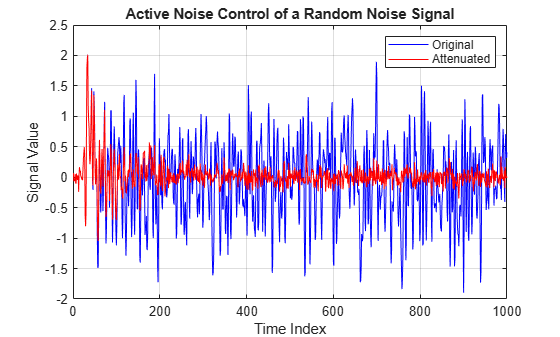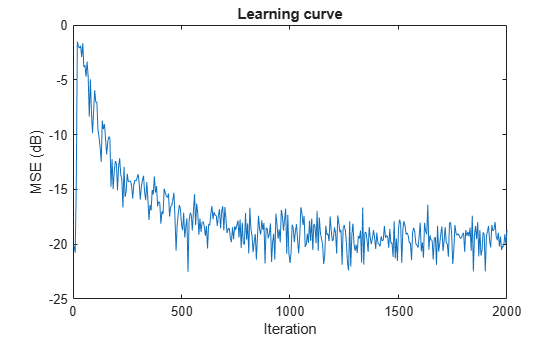Main Content

# dsp.FilteredXLMSFilter

Filtered XLMS filter

## Description

The `dsp.FilteredXLMSFilter` System object™ computes output, error and coefficients using filtered-x least mean square FIR adaptive filter.

To implement the adaptive FIR filter object:

1. Create the `dsp.FilteredXLMSFilter` object and set its properties.

2. Call the object with arguments, as if it were a function.

To learn more about how System objects work, see What Are System Objects?.

## Creation

### Syntax

``fxlms = dsp.FilteredXLMSFilter``
``fxlms = dsp.FilteredXLMSFilter(len)``
``fxlms = dsp.FilteredXLMSFilter(Name,Value)``

### Description

````fxlms = dsp.FilteredXLMSFilter` returns a filtered-x least mean square FIR adaptive filter System object, `fxlms`. This System object is used to compute the filtered output and the filter error for a given input and desired signal.```

example

````fxlms = dsp.FilteredXLMSFilter(len)` returns a `FilteredXLMSFilter` System object, `fxlms`, with the `Length` property set to `len`.```

example

````fxlms = dsp.FilteredXLMSFilter(Name,Value)` returns a `FilteredXLMSFilter` System object, `fxlms`, with each specified property set to the specified value. Enclose each property name in single quotes. Unspecified properties have default values.```

## Properties

expand all

Unless otherwise indicated, properties are nontunable, which means you cannot change their values after calling the object. Objects lock when you call them, and the `release` function unlocks them.

If a property is tunable, you can change its value at any time.

For more information on changing property values, see System Design in MATLAB Using System Objects.

Specify the length of the FIR filter coefficients vector as a positive integer value. This property is nontunable.

Data Types: `single` | `double` | `int8` | `int16` | `int32` | `int64` | `uint8` | `uint16` | `uint32` | `uint64`

Specify the adaptation step size factor as a positive numeric scalar.

Tunable: Yes

Data Types: `single` | `double` | `int8` | `int16` | `int32` | `int64` | `uint8` | `uint16` | `uint32` | `uint64`

Specify the leakage factor used in a leaky adaptive filter as a numeric value between `0` and `1`, both inclusive. When the value is less than `1`, the System object implements a leaky adaptive algorithm. The default value is `1`, providing no leakage in the adapting method.

Tunable: Yes

Data Types: `single` | `double` | `int8` | `int16` | `int32` | `int64` | `uint8` | `uint16` | `uint32` | `uint64` | `logical`

Specify the coefficients of the secondary path filter model as a numeric vector. The secondary path connects the output actuator and the error sensor. The default value is a vector that represents the coefficients of a 10th-order FIR lowpass filter.

Tunable: Yes

Data Types: `single` | `double` | `int8` | `int16` | `int32` | `int64` | `uint8` | `uint16` | `uint32` | `uint64`

Specify the estimate of the secondary path filter model as a numeric vector. The secondary path connects the output actuator and the error sensor. The default value equals to the `SecondayPathCoefficients` property value. This property is not tunable.

Data Types: `single` | `double` | `int8` | `int16` | `int32` | `int64` | `uint8` | `uint16` | `uint32` | `uint64`

Specify the initial values of the FIR adaptive filter coefficients as a scalar or a vector of length equal to the value of the `Length` property.

Tunable: Yes

Data Types: `single` | `double` | `int8` | `int16` | `int32` | `int64` | `uint8` | `uint16` | `uint32` | `uint64`

Specify whether to lock the filter coefficient values. By default, the value of this property is `false`, and the object continuously updates the filter coefficients. If this property is set to `true`, the filter coefficients do not update and their values remain the same.

Tunable: Yes

## Usage

### Syntax

``[y,err] = fxlms(x,d)``

### Description

example

````[y,err] = fxlms(x,d)` filters the input `x`, using `d` as the desired signal, and returns the filtered output `y` and the filter error `err`. The System object estimates the filter weights needed to minimize the error between the output signal and the desired signal. You can access these coefficients by accessing the `Coefficients` property of the object. This can be done only after calling the object. For example, to access the optimized coefficients of the `fxlms` filter, call `fxlms.Coefficients` after you pass the input and desired signal to the object.```

### Input Arguments

expand all

The signal to be filtered by the filtered XLMS filter. The input, `x`, and the desired signal, `d`, must have the same size and data type.

The input, `x` can be a variable-size signal. You can change the number of elements in the column vector even when the object is locked. The System object locks when you call the object to run its algorithm.

Data Types: `single` | `double`
Complex Number Support: Yes

The filtered XLMS filter adapts its coefficients to minimize the error, `err`, and converge the input signal `x` to the desired signal `d` as closely as possible.

The input, `x`, and the desired signal, `d`, must have the same size and data type.

The desired signal, `d`, can be a variable-size signal. You can change the number of elements in the column vector even when the object is locked. The System object locks when you call the object to run its algorithm.

Data Types: `single` | `double`
Complex Number Support: Yes

### Output Arguments

expand all

Filtered output, returned as a scalar or a column vector. The object adapts its filter coefficients to converge the input signal `x` to match the desired signal `d`. The filter outputs the converged signal.

Data Types: `single` | `double`
Complex Number Support: Yes

Difference between the output signal `y` and the desired signal `d`, returned as a scalar or a column vector. The objective of the filtered XLMS filter is to minimize this error. The object adapts its coefficients to converge towards optimal filter coefficients that produce an output signal that matches closely with the desired signal. To access the filtered XLMS filter coefficients, call `fxlms.Coefficients` after you pass the input and desired signal to the object.

Data Types: `single` | `double`
Complex Number Support: Yes

## Object Functions

To use an object function, specify the System object as the first input argument. For example, to release system resources of a System object named `obj`, use this syntax:

`release(obj)`

expand all

 `msesim` Estimated mean squared error for adaptive filters
 `step` Run System object algorithm `release` Release resources and allow changes to System object property values and input characteristics `reset` Reset internal states of System object

## Examples

collapse all

Note: If you are using R2016a or an earlier release, replace each call to the object with the equivalent `step` syntax. For example, `obj(x)` becomes `step(obj,x)`.

Generate noise, create FIR primary path system model, generate observation noise, filter the primary path system model output with added noise, and create FIR secondary path system model.

```x = randn(1000,1); g = fir1(47,0.4); n = 0.1*randn(1000,1); d = filter(g,1,x) + n; b = fir1(31,0.5);```

Use the `dsp.FilteredXLMSFilter` System object™ to compute the filtered output and the filter error for the input and the signal to be canceled.

```mu = 0.008; fxlms = dsp.FilteredXLMSFilter(32, 'StepSize', mu, 'LeakageFactor', ... 1, 'SecondaryPathCoefficients', b); [y,e] = fxlms(x,d);```

Plot the results.

```plot(1:1000,d,'b',1:1000,e,'r'); title('Active Noise Control of a Random Noise Signal'); legend('Original','Attenuated'); xlabel('Time Index'); ylabel('Signal Value'); grid on;```Identify an unknown system by performing active noise control using a filtered-x LMS algorithm. The objective of the adaptive filter is to minimize the error signal between the output of the adaptive filter and the output of the unknown system (or the system to be identified). Once the error signal is minimal, the unknown system converges to the adaptive filter.

Note: If you are using R2016a or an earlier release, replace each call to the object with the equivalent step syntax. For example, `obj(x)` becomes `step(obj,x)`.

Initialization

Create a `dsp.FIRFilter` System object that represents the system to be identified. Pass the signal, `x`, to the FIR filter. The output of the unknown system is the desired signal, `d`, which is the sum of the output of the unknown system (FIR filter) and an additive noise signal, `n`.

```num = fir1(31,0.5); fir = dsp.FIRFilter('Numerator',num); iir = dsp.IIRFilter('Numerator',sqrt(0.75),... 'Denominator',[1 -0.5]); x = iir(sign(randn(2000,25))); n = 0.1*randn(size(x)); d = fir(x) + n; ```

Adaptive Filter

Create a `dsp.FilteredXLMSFilter` System object to create an adaptive filter that uses the filtered-x LMS algorithm. Set the length of the adaptive filter to 32 taps, step size to 0.008, and the decimation factor for analysis and simulation to 5. The variable `simmse` represents the error between the output of the unknown system, `d`, and the output of the adaptive filter.

```l = 32; mu = 0.008; m = 5; fxlms = dsp.FilteredXLMSFilter(l,'StepSize',mu); [simmse,meanWsim,Wsim,traceKsim] = msesim(fxlms,x,d,m); plot(m*(1:length(simmse)),10*log10(simmse)) xlabel('Iteration') ylabel('MSE (dB)') title('Learning curve for Filtered-x LMS filter used in system identification')```With each iteration of adaptation, the value of `simmse` decreases to a minimal value, indicating that the unknown system has converged to the adaptive filter.

 Kuo, S.M. and Morgan, D.R. Active Noise Control Systems: Algorithms and DSP Implementations. New York: John Wiley & Sons, 1996.

 Widrow, B. and Stearns, S.D. Adaptive Signal Processing. Upper Saddle River, N.J: Prentice Hall, 1985.

Watch now